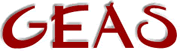[ < ] [ > ] [ << ] [ Up ] [ >> ] [Top] [Contents] [Index] [ ? ]

### 1.2.8 Prefix allocation

All of the arithmetic and boolean operator expressions can be written in a shorter way if all you want to do is compute one variable with any other expression and then store the result in the variable again.

Say that what you want to do is this `a = a + 5;`, a much neater way of writing that is `a += 5;`. The value of the second expression is added to the first and then stored in the first which happens to be a variable.

You write all the others in the same way, i.e. the variable, then the operator directly followed by `=` and then the expression.

 ```a >>= 5; // a = a >> 5; b %= d + 4; // b = b % (d + 4); c ^= 44 & q; // c = c ^ (44 & q); ```

This document was generated by Ronny Wikh on July, 8 2003 using texi2html